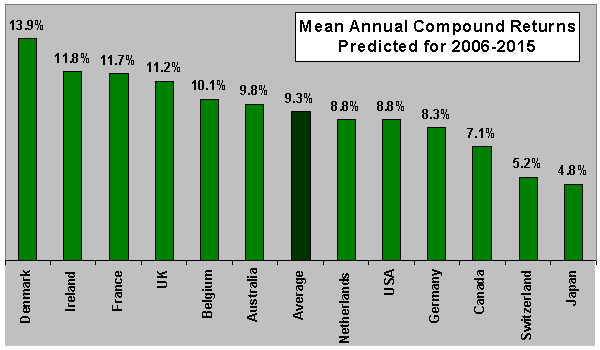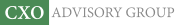Objective research to aid investing decisions

#### Value Investing Strategy (Strategy Overview)

Allocations for October 2023 (Final)
Cash TLT LQD SPY

#### Momentum Investing Strategy (Strategy Overview)

Allocations for October 2023 (Final)
1st ETF 2nd ETF 3rd ETF

# Worldwide Equity Returns in the 21st Century

| | Posted in: Equity Premium

In his June 2006 article entitled “Investing in the 21st Century: With Occam’s Razor and Bogle’s Wit”, Javier Estrada evaluates the long-term forecasting abilities of two simple models over 10-year periods during 1973-2005. He then uses them to predict the returns for 12 country stock markets (Australia, Belgium, Canada, Denmark, France, Germany, Ireland, Japan, Netherlands, Switzerland, UK, USA) for 2006-2015. He finds that:

The author’s Returns Decomposition Model considers the following four variables: the initial dividend yield; the expected growth of dividends; the expected growth of earnings; and, the expected change in the price-earnings ratio (P/E). It assumes that the mean annual compound growth rates for earnings and dividends over each forecasted 10-year period revert to the rates experienced from 1973 to the beginning of each prediction period. For P/E, it addresses three scenarios: (1) P/E at the end of each forecasted 10-year period reverts to the average P/E between 1973 and the beginning of each prediction period; (2) P/E remains constant at each initial value throughout the prediction period; and, (3) P/E at the end of each forecasted period is the average of the mean P/E over the five years before the prediction is made, and the mean P/E between 1973 and the beginning of those five years.

The author’s Price Decomposition Model simplifies the above approach, using only the expected growth of earnings; and, the expected change in P/E to predict equity returns.

• The Returns Decomposition Model produces somewhat more accurate in-sample forecasts than does the Price Decomposition Model.
• Forecasts assuming the mean reversion of P/E produce higher correlations with actual data, but also higher mean forecast errors and higher mean absolute forecast errors.
• The models work well in some countries (including the U.S.), but poorly in others (such as Japan).
• The nominal mean annual compound rates of return for the 12 countries predicted over 2006-2015 range from 4.8% (Japan) to 13.9% (Denmark), with an average of 9.3%. The expectation for the U.S. is 8.8%.

The following chart, constructed from data in the paper, shows the nominal mean annual compound rates of return predicted over 2006-2015 for all 12 countries.In summary, the author forecasts annual worldwide equity returns in the high single digits over the coming decade.